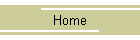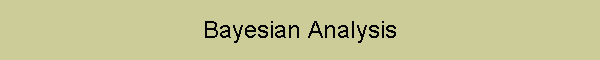ECHONOMY
Tools for Echocardiographic Calculations

Muhamed Saric, MD, PhD
New York University## Purpose

To demonstrate the impact of disease prevalence (pretest probability) on positive and negative predictive values given the sensitivity and the specificity of the test. Although the calculator is used in the context of stress testing for coronary artery disease, basic principles apply to any testing.

## Major Points

No matter how good an interpreting physician is, some stress test results will be false positive and some false negative. The primary determinant of the number of falsely read stress tests is the prevalence of the coronary artery disease (CAD) in the test population.

## Calculator

Disease prevalence
(pretest probability of disease)

%

Low: <10%
Moderate: 20 - 30%
High: >30%

Sensitivity

%

XSE = 74 - 97% (mean 88%)
DSE = 61 - 95% (mean 81%)
PSE = 61 - 81% (mean 77%)

Specificity

%

XSE = 64 - 86% (mean 79%)
DSE = 51 - 95% (mean 80%)
PSE = 90 - 94% (mean 92%)
XSE, exercise stress echo
DSE, dobutamine stress echo
PSE, persantine stress echo

Positive predictive value (PPV)

%

Negative  predictive value (NPV)

%

False positives  %
False negatives  %

## Interpretation

PPV: Proportion of all positive stress tests that are truly positive, i.e. likelihood that a positive stress test is correct in identifying the disease.

NPV: Proportion of all negative stress tests are truly negative; i.e. likelihood that a negative stress test is correct in ruling out the disease.

Stress testing works best when the prevalence (pretest probability) of CAD is moderate (10 - 30%). In low-risk populations, there is a large number of false positives and only a few false negatives. In high-risk populations, there is a large number of false negatives and a few false positives.

## Formula

Sensitivity = TP / P = TP / (TP + FN)

Specificity = TN / (1 - P) = TN / (TN + FP)

PPV = TP / (TP + FP)

NPV = TN / (TN + FN)

Abbreviations: TP, true positives; FP, false positives; TN, true negatives; FN, false negatives; P, prevalence (pretest probability).

## Reference

Kline JA, Johnson CL, Pollack CV Jr, Diercks DB, Hollander JE, Newgard CD, Garvey JL. Pretest probability assessment derived from attribute matching. BMC Med Inform Decis Mak. 2005 Aug 11;5:26.

Marwick TH. Stress echocardiography. Heart. 2003 Jan;89(1):113-8.